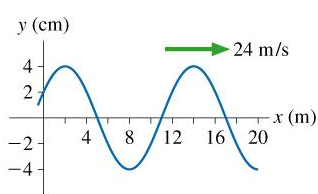# Problem: The figure is a snapshot graph of a wave at t=0s; A=4.0cm; Wavelength=12 mWhat is the frequency of this wave?(Hz)

###### FREE Expert Solution

Frequency, f = v/λ

From the graph, λ = 14 - 2 = 12m

95% (5 ratings)###### Problem DetailsThe figure is a snapshot graph of a wave at t=0s; A=4.0cm; Wavelength=12 m
What is the frequency of this wave?(Hz)